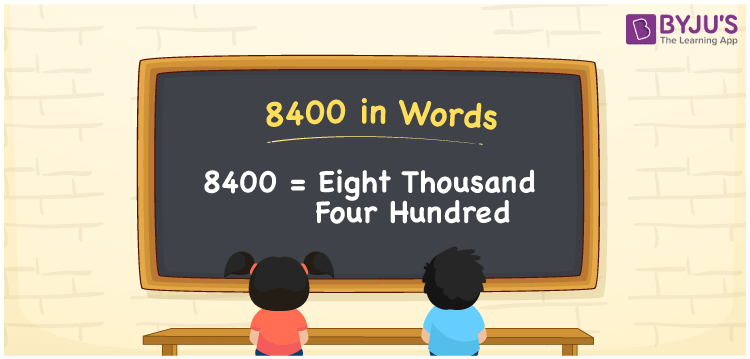# 8400 in Words

8400 in words is written as Eight thousand four hundred. In both the International System of Numerals and the Indian System of Numerals, 8400 is written as Eight thousand four hundred. The number 8400 is a Cardinal Number as it represents some quantity. For example, “Mary’s total expenditure for the month of April was 8400 rupees”.

 8400 in words Eight thousand four hundred Eight thousand four hundred in Number 8400

## 8400 in English Words

8400 in English words is read as “Eight thousand four hundred.”## How to Write 8400 in Words?

To write 8400 in words, we shall use the place value chart. In the place value chart, put 8 in the thousands, 4 in the hundreds, and 0 in the tens and the ones place respectively. Let us make a place value chart to write the number 8400 in words.

 Thousands Hundreds Tens Ones 8 4 0 0

Thus, we can write the expanded form as

8 × Thousand + 4 × Hundred + 0 × Ten + 0 × One

= 8 × 1000 + 4 × 100 + 0 × 10 + 0 × 1

= 8000 + 400 + 0 + 0

= 8400

= Eight thousand four hundred.

8400 is the natural number that is succeeded by 8399 and preceded by 8401.

8400 in words – Eight thousand four hundred

• Is 8400 an odd number? – No
• Is 8400 an even number? – Yes
• Is 8400 a perfect square number? – No
• Is 8400 a perfect cube number? – No
• Is 8400 a prime number? – No
• Is 8400 a composite number? – Yes

## Frequently Asked Questions on 8400 in Words

Q1

### How to write 8400 in words?

8400 in words is written as Eight thousand four hundred.
Q2

### How to write 8400 in words in the International and Indian System of Numerals?

In both, the system of numerals, 8400 in words, is written as Eight thousand four hundred.
Q3

### How to write 8400 in a place value chart?

In the place value chart, write 8 in the thousands, 4 in the hundreds, and 0 in the tens and the ones, respectively.